Let us analyze the following situation for a better understanding.

Drag the slider to change the angle of the car's position with reference to its starting point on the x-axis.

The angle's measurement in degrees is changing with the car's respective position as it moves forward.

What are the different units to represent this measurement? Let's explore this through the simulation and then read the lesson to learn about radian.

Do you know that radian was the SI supplementary unit for measuring angles before 1995? It was later changed to a derived unit. In this mini-lesson, we will explore the world of converting degrees to radians using interactive simulations. We will also cover the various concepts on the subject including converting degrees to radians formula, converting degrees to radians calculator, converting degrees to radians worksheet, degrees to radians chart, and degrees to radians equation.

Do not forget to try your hand at solving a few interesting practice questions at the end of the page.

## Explain Degrees

A degree, which is referred to as the degree of arc or arc degree, is the unit of measuring a plane angle.

It is denoted by the symbol $$(^\circ)$$.

A complete rotation is denoted by the angle measuring $$360^\circ$$ and the device used to measure an angle in degrees is known as the protractor.

The following figure shows different scales of measuring an angle in degrees: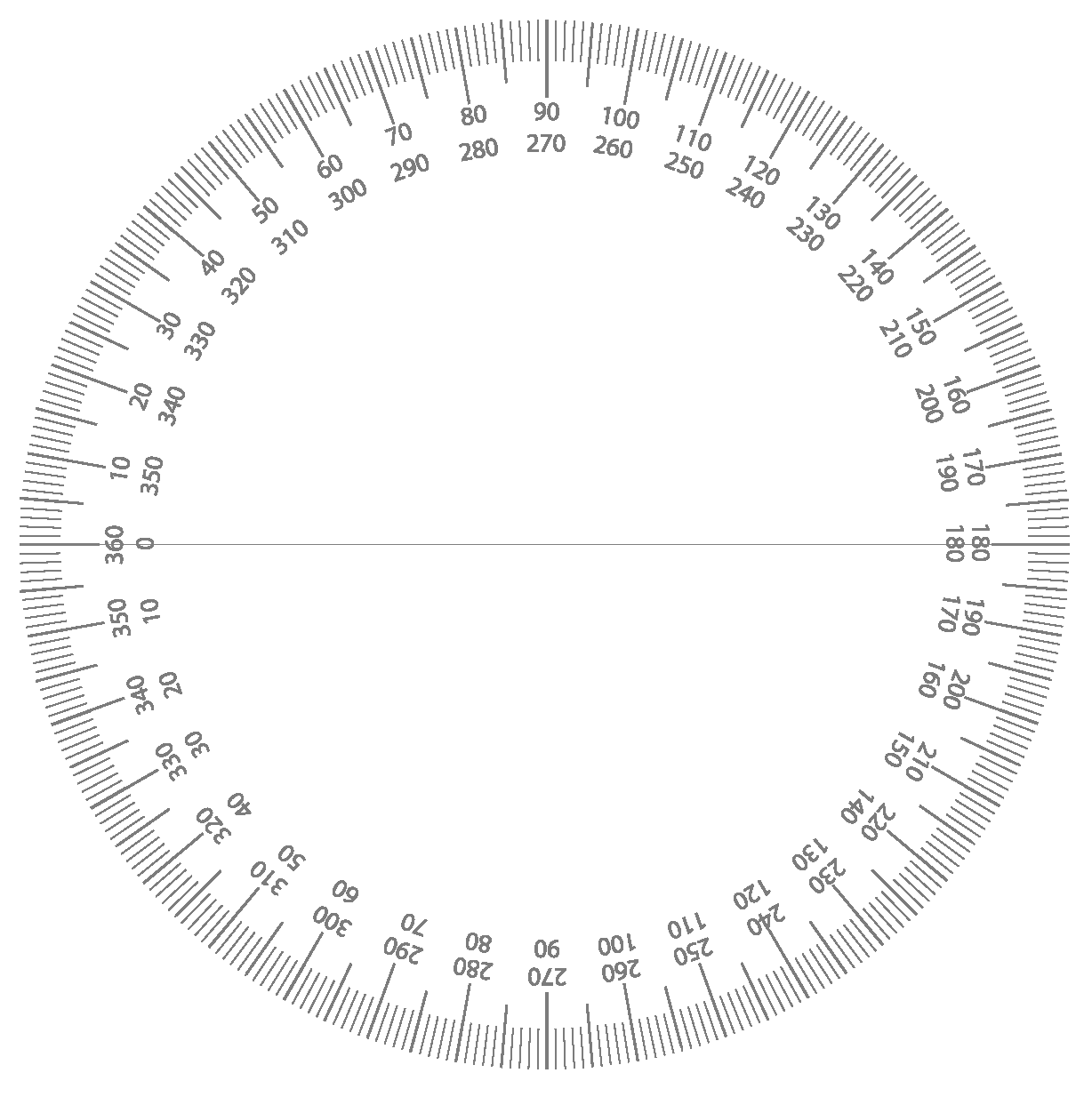Radian is another unit of measuring an angle which is made by wrapping a radius along the circle.

One Radian is $$\frac{180}{\pi}$$ degrees, which is equal to about 57.2958 degrees.

One complete counterclockwise revolution, in radians, is equal to $$2 \pi$$.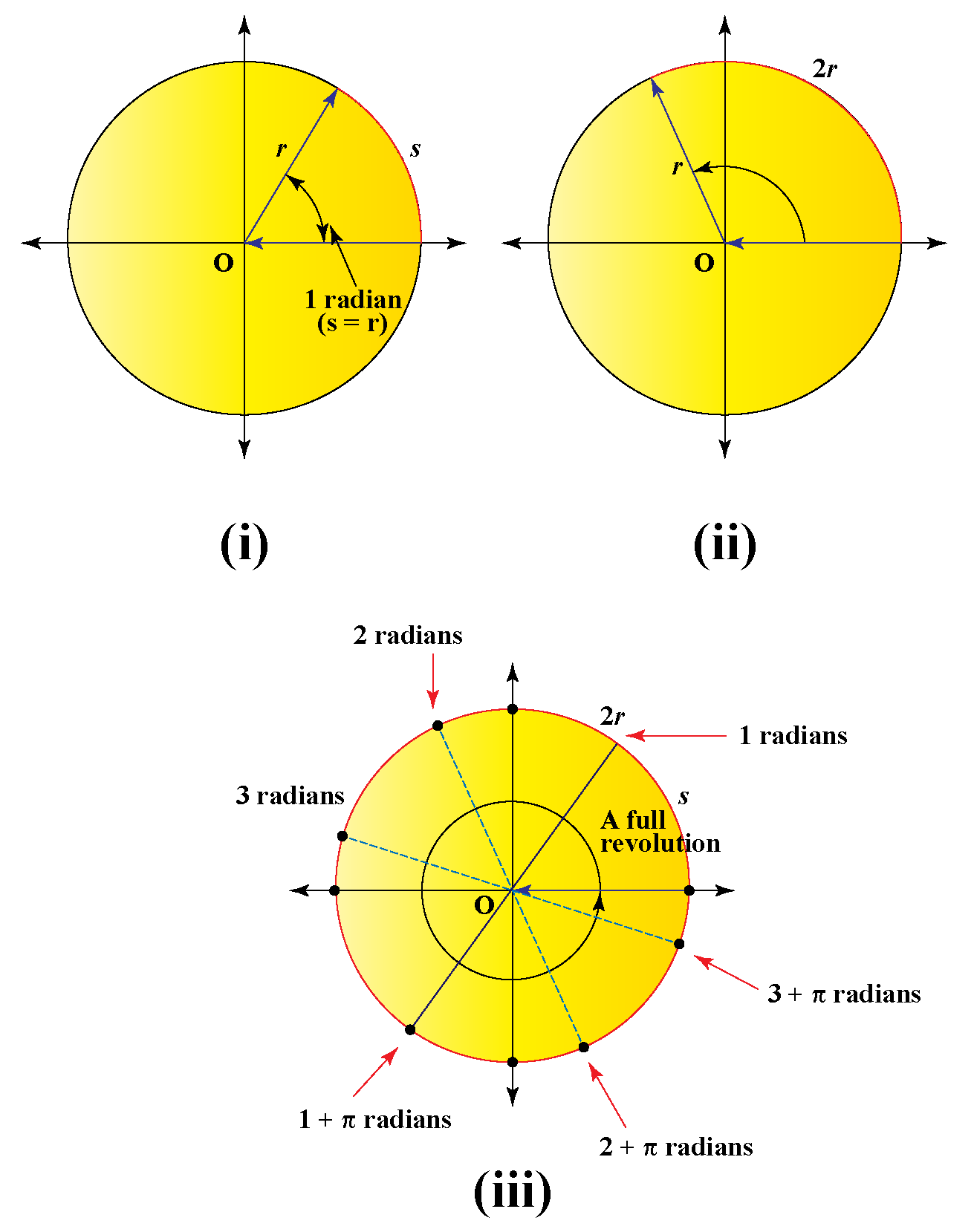## How to Convert Degrees to Radians?

The amount of rotation from the starting side to the terminal side determines the measure of an angle.

One complete counterclockwise revolution in radians is equal to $$2 \pi$$.

Similarly, in degrees, one complete counterclockwise revolution is $$360^\circ$$.

Comparing both the values, we get,

\begin{align*} 360^\circ &= 2 \pi \\ 1^\circ &= \frac{360}{2 \pi} \\ 1^\circ &= \frac{180}{\pi} \end{align*}

Thus, to convert degrees to radians, we use the formula:

 Radians = Degrees $$\times \frac{\pi}{180}$$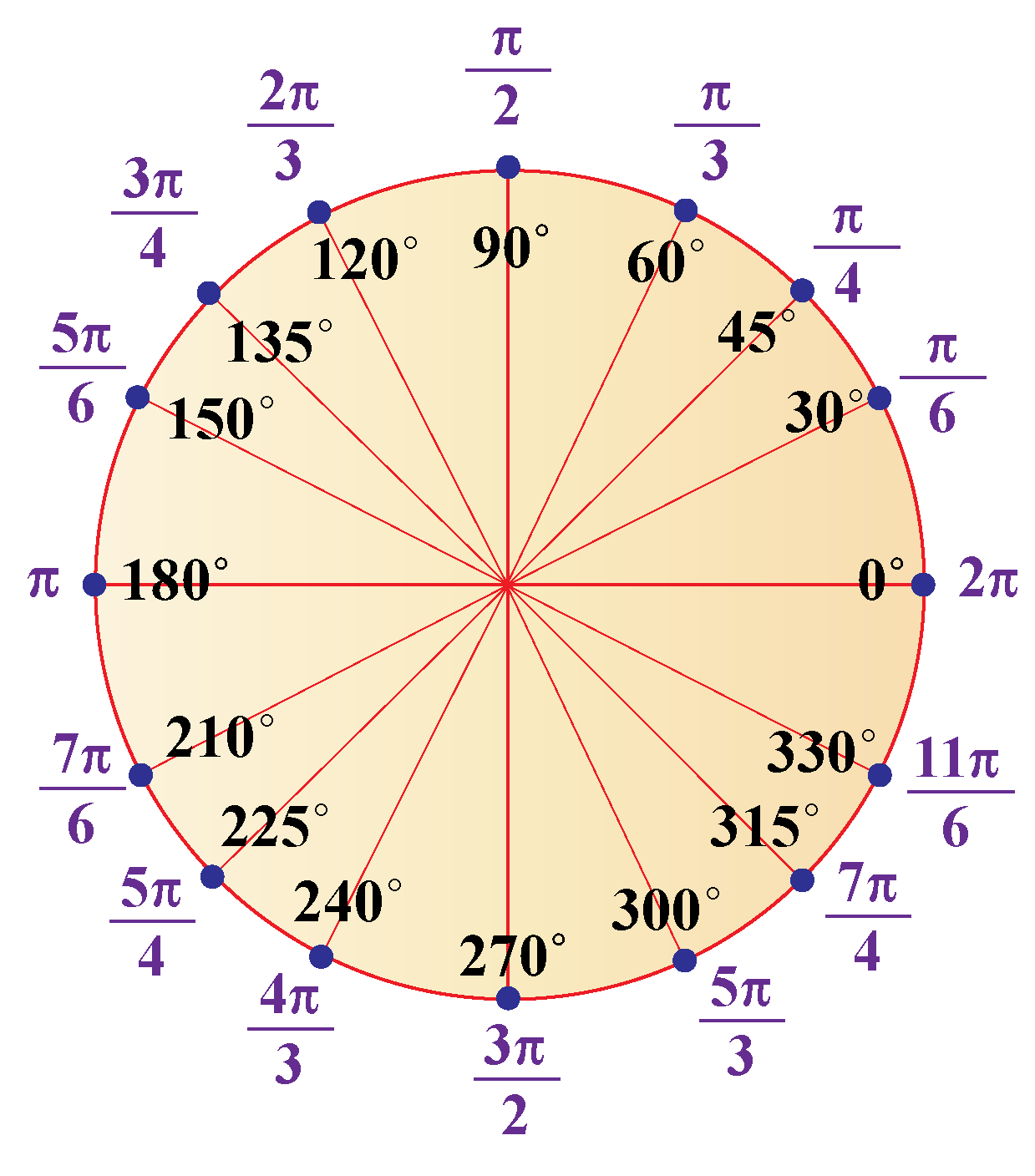Think Tank
• How would you experimentally calculate the value of $$\pi$$ using a given circle?

## Degrees to Radians Conversion Calculator

Check out this degrees to radians conversion calculator to see how degrees are converted to radian.

## Degrees to Radians Conversion Table

You can refer to the following table to see the radian value of some angles given in degrees:

$$0^\circ$$ $$0$$ rad
$$30^\circ$$ $$\frac{ \pi }{ 6}$$ rad
$$45^\circ$$ $$\frac{ \pi }{ 4}$$ rad
$$60^\circ$$ $$\frac{ \pi }{ 3}$$ rad
$$90^\circ$$ $$\frac{ \pi }{ 2}$$ rad
$$120^\circ$$ $$\frac{ 2 \pi }{ 3}$$ rad
$$135^\circ$$ $$\frac{ 3 \pi }{ 4}$$ rad
$$150^\circ$$ $$\frac{ 5 \pi }{ 6}$$ rad
$$180^\circ$$ $$\pi$$ rad
$$270^\circ$$ $$\frac{ 3 \pi }{ 2}$$ rad
$$360^\circ$$ $$2 \pi$$ radChallenging Question
• A certain line from the origin divides the third quadrant into two equal sections. What will be the anti-clockwise angle subtended between this line and the reference line in radians?

## Solved Examples

 Example 1

In a circle with center O, points A and B lie on the circle. $$\angle{AOB }$$ has a measure of $$60^\circ$$. What is the radian measure of $$\angle{AOB}$$?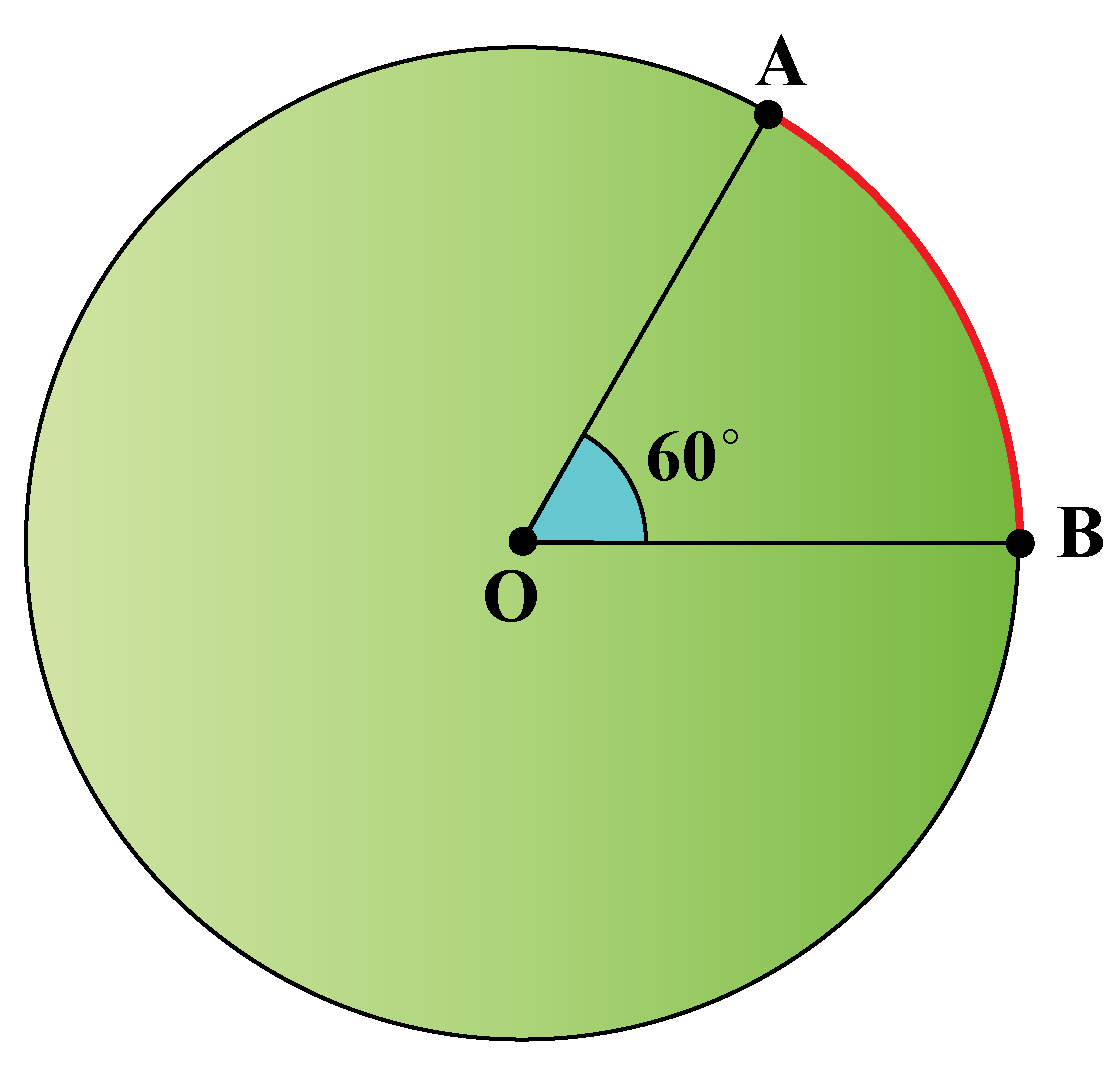Solution

We have,

$$\angle{AOB} = \frac{\pi}{3}$$

Degrees to radians conversion formula is given as,

Radians = Degrees $$\times \frac{\pi}{180}$$

Thus converting the given angle from degrees to radians, we get,

\begin{align*} \angle{AOB} \text { in radians} &= \angle{AOB} \text { in degrees} \times \frac{\pi}{180} \\ \angle{AOB} &= 60 \times \frac{\pi}{180} \\ &= \frac{\pi}{3} \end{align*}

Hence,

$$\angle{AOB} = \frac{\pi}{3} \ \text {rad}$$

 $$\therefore$$ $$\angle{AOB} = \frac{\pi}{3} \ \text {rad}$$
 Example 2

Katie bought a pizza from a nearby store. Her mother gave her a slice which measured $$\frac{1}{4}^ \text {th}$$ of the complete pizza.

Can you help Katie find the measurement of the angle in degree and radian?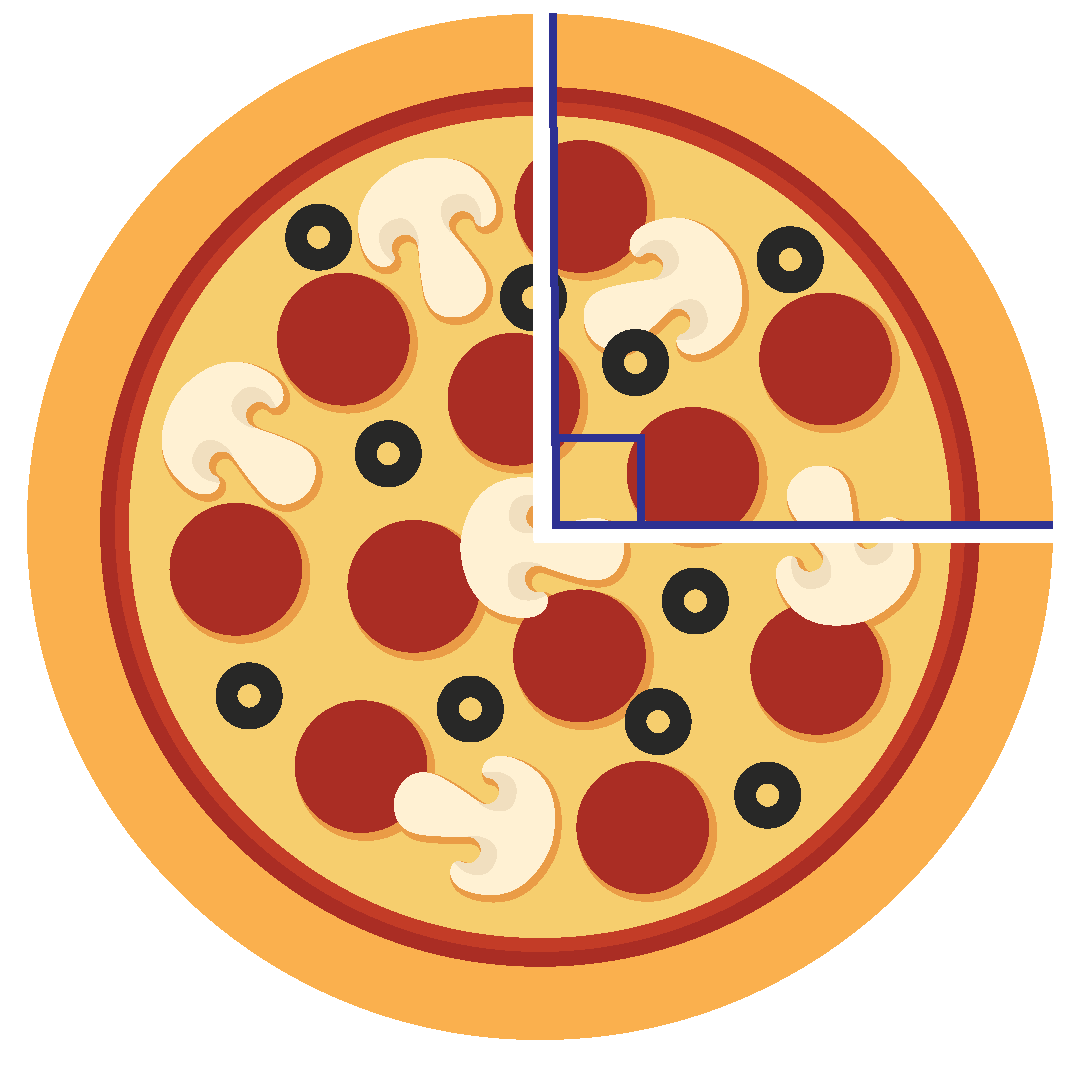Solution

Here,

The value portion Katie got = $$\frac{1}{4}^ \text {th}$$ of the complete pizza.

Since the shape of a pizza is circular, and the angle for a complete circle = $$360^\circ$$, thus we can conclude that:

Angle for the complete pizza = $$360^\circ$$

The angle for the $$\frac{1}{4}^ \text {th}$$ slice = $$\frac{360^\circ}{4} = 90^\circ$$

We know,

The value of this angle in radians = value in degrees $$\times \frac{\pi}{180}$$

Thus,

The angle of Katie's slice in radians = $$90^\circ \times \frac{\pi}{180} = \frac{\pi}{2}$$

 $$\therefore$$ The angle of Katie's slice = $$90^\circ = \frac{\pi}{2}$$

## Interactive Questions

Here are a few activities for you to practice. Select/Type your answer and click the "Check Answer" button to see the result.

## Let's Summarize

The mini-lesson targeted the fascinating concept of converting degrees to radians. The math journey around converting degrees to radians starts with what a student already knows, and goes on to creatively crafting a fresh concept in the young minds. Done in a way that not only it is relatable and easy to grasp, but also will stay with them forever. Here lies the magic with Cuemath.

At Cuemath, our team of math experts is dedicated to making learning fun for our favorite readers, the students!

Through an interactive and engaging learning-teaching-learning approach, the teachers explore all angles of a topic.

Be it worksheets, online classes, doubt sessions, or any other form of relation, it’s the logical thinking and smart learning approach that we, at Cuemath, believe in.

## 1. Explain the radian of a circle?

Radian is another unit for measuring angles. It is the angle made by wrapping a radius along the circle.

One radian is $$\frac{180}{\pi}$$ degrees, which is equal to about 57.2958 degrees.

One complete counterclockwise revolution in radians is equal to $$2 \pi$$

More Important Topics
Numbers
Algebra
Geometry
Measurement
Money
Data
Trigonometry
Calculus